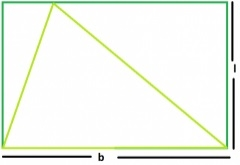# Area of the largest triangle that can be inscribed within a rectangle?

A rectangle is a quadrilateral that has opposite side equal and parallel. The adjacent side are at 90o. And a triangle is a closed figure with three sides.

The largest triangle inscribed within a rectangle. Has its base equal to the length of the rectangle and height of the triangle is equal to the breadth of the rectangle.Area = (½)*l*b

Area of largest triangle inscribed in a rectangle = (½)*l*b

Program to calculate the area of the largest triangle inscribed in a rectangle −

## Example Code

#include <stdio.h>
int main(void) {
int l = 10, b = 9;
float area ;
area = (float)((l*b)/2);
printf("Area of largest triangle inscribed in a rectangle of
length %d and breadth %d is %f",l,b,area);
return 0;
}

## Output

Area of largest triangle inscribed in a rectangle of length 10 and breadth 9 is 45.000000